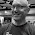## Friday, December 14, 2018

### LSM math - size of search space for LSM tree configuration

I have written before and will write again about using 3-tuples to explain the shape of an LSM tree. This makes it easier to explain the configurations supported today and configurations we might want to support tomorrow in addition to traditional tiered and leveled compaction. The summary is that n LSM tree has N levels labeled from L1 to Ln and Lmax is another name for Ln. There is one 3-tuple per level and the components of the 3-tuple are (type, fanout, runs) for Lk (level k) where:
• type is Tiered or Leveled and explains compaction into that level
• fanout is the size of a sorted run in Lk relative to a sorted run from Lk-1, a real and >= 1
• runs is the number of sorted runs in that level, an integer and >= 1
Given the above how many valid configurations exist for an LSM tree? There are additional constraints that can be imposed on the 3-tuple but I will ignore most of them except for limiting fanout and runs to be <= 20. The answer is easy - there are an infinite number of configurations because fanout is a real.

The question is more interesting when fanout is limited to an integer and the number of levels is limited to between 1 and 10. I am doing this to explain the size of the search space but I don't think that fanout should be limited to an integer.

There are approximately 2^11 configurations only considering compaction type, which has 2 values, and 1 to 10 levels because there are 2^N configurations of compaction types for a tree with N levels and the sum of 2^1 + 2^2 + ... + 2^9 + 2^10 = 2^11 - 1

But when type, fanout and runs are considered then there are 2 x 20 x 20 = 800 choices per level and 800^N combinations for an LSM tree with N levels. Considering LSM trees with 1 to 10 levels then the number of valid configurations is the sum 800^1 + 800^2 + ... + 800^9 + 800^10. That is a large number of configurations if exhaustive search were to be used to find the best configuration. Note that I don't think exhaustive search should be used.

1.1.